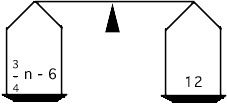hello, I am in 6th grade advanced math and I just started Algebra. My teacher tries to explain it to me but I just can't get it. I am having difficulty understanding it. Please HELP!!! I will type a problem, if you could answer it and show your work so i can understand it that would be great. Please explain in simple terms. here is a couple problems I am having trouble with, PROBLEM #1 3/4n-6=12 n= Problem #2 1 3/4x+6=20 x= Thank you, Person Seeking Help Hi, It helps some times to think of an equation as a scale that has a pan for weights on each side. In your problem #1 the sacle is balanced with a weight of  3/4 n - 6 on one side and 12 on the other.The task is to move the weights around, always maintaining the balance, and end with one side having only n on it. The balance is maintained if you add the same amount to each side, subtract the same amount from each side, multiply each side by the same amount or divide each side by the same amount. Lets start with problem #1. The left side has  3/4 n - 6 on it and I want only n on this side, so the first step I would take is to add 6 to each side. This will maintain the balance and eliminate the - 6 from the left side.Thus  3/4 n - 6 = 12 becomes  3/4 n - 6 + 6 = 12 + 6 or  3/4 n = 18 Now multiply each side by 4 to get 4( 3/4 n) = 4(18) which is then 3 n = 72 Finally divide both sides by 3 to get  (3 n)/3 = 72/3 which is n = 24 You should check your answer by calculating  3/4 n - 6 when n is 24 to see that you get 12. Problem #2 is similar with one extra complication. In this problem you have 1 3/4 as the coefficient of x. Before you start through the steps above you should write 1 3/4 as a common fraction rather than a mixed fraction. That is write 1 3/4 as  7/4. Now go through steps similar to what I did above to get x alone on the left side. Let me know what you find for x. Penny Go to Math Central